# Relation between gradient vector and directional derivatives

at a point, in vector notation (multiple variables) Suppose$f$ is a function of a vector variable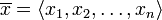$\overline{x} = \langle x_1,x_2,\dots,x_n \rangle$. Suppose$\overline{u}$ is a unit vector and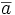$\overline{a}$ is a point in the domain of$f$. Suppose that the gradient vector of$f$ at$\overline{a}$ exists. We denote this gradient vector by$\nabla f(\overline{a})$. Then, we have the following relationship: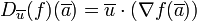$D_{\overline{u}}(f)(\overline{a}) = \overline{u} \cdot (\nabla f(\overline{a}))$
generic point, in vector notation (multiple variables) Suppose$f$ is a function of a vector variable$\overline{x} = \langle x_1,x_2,\dots,x_n \rangle$. Suppose$\overline{u}$ is a unit vector. We then have: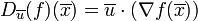$D_{\overline{u}}(f)(\overline{x}) = \overline{u} \cdot (\nabla f(\overline{x}))$
generic point, point-free notation (multiple variables) Suppose$f$ is a function of a vector variable$\overline{x} = \langle x_1,x_2,\dots,x_n \rangle$. Suppose$\overline{u}$ is a unit vector. We then have: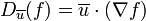$D_{\overline{u}}(f) = \overline{u} \cdot (\nabla f)$
The right side here is a dot product of vector-valued functions (the constant function$\overline{u}$ and the gradient vector of$f$). The equality holds whenever the right side makes sense.